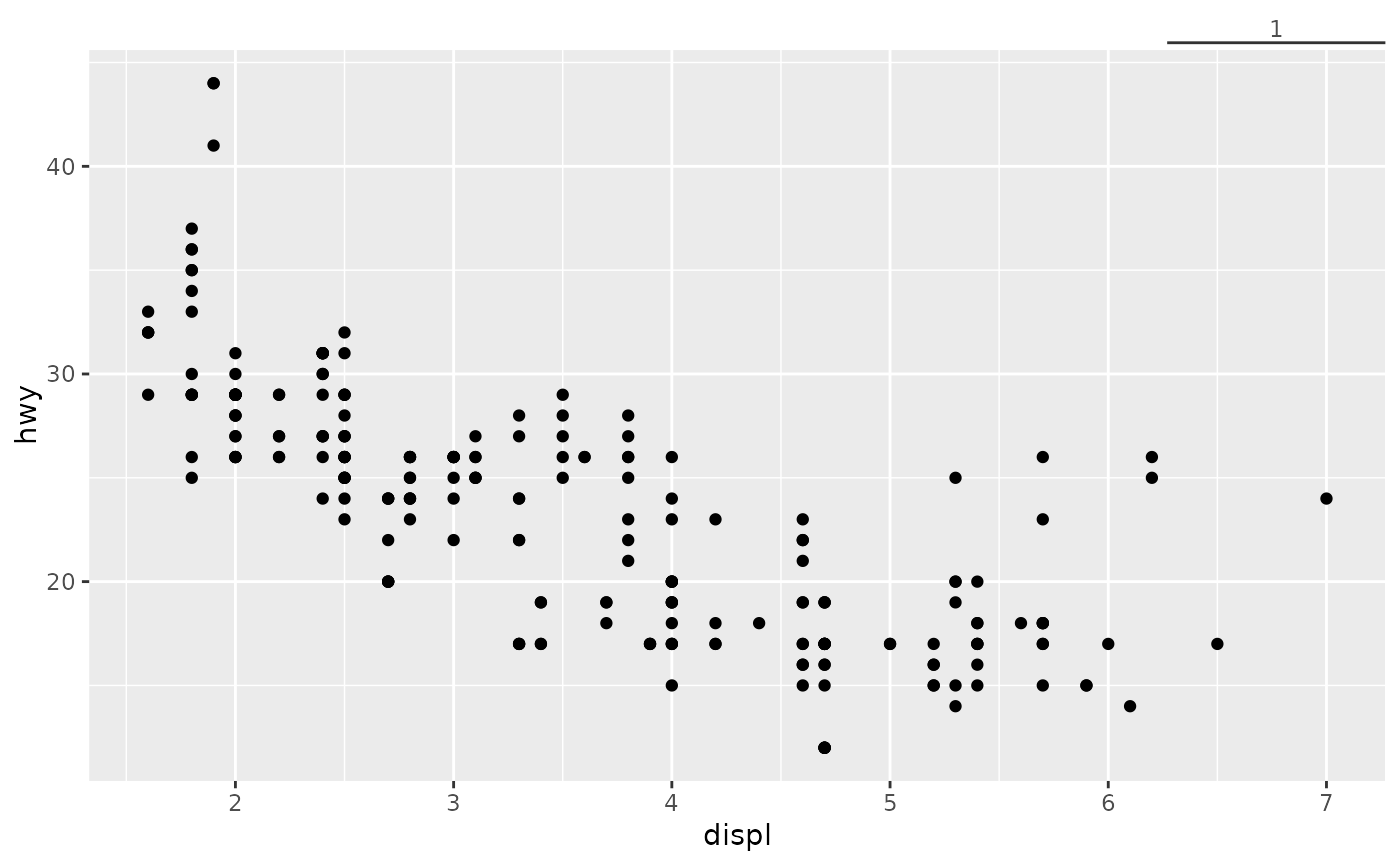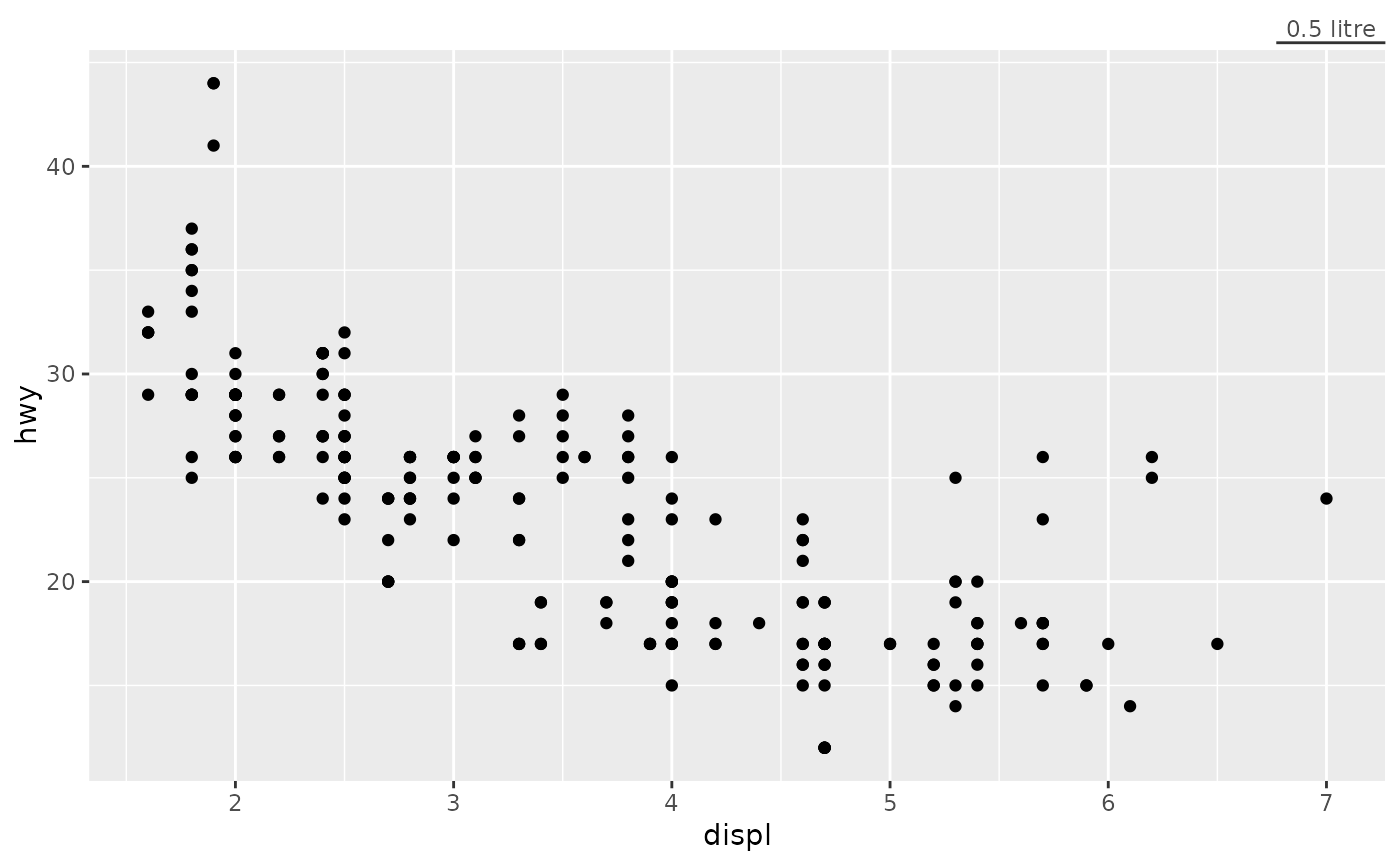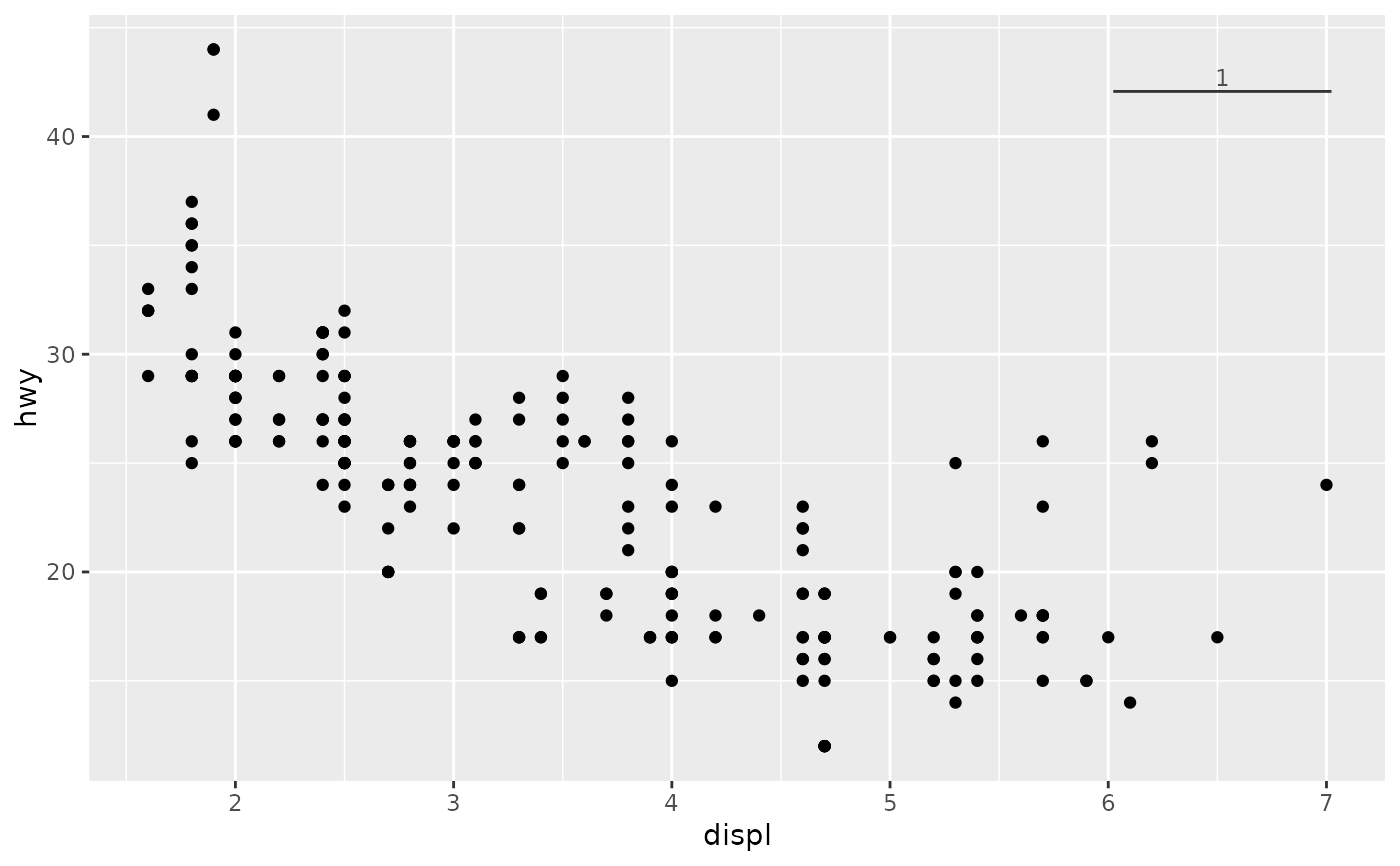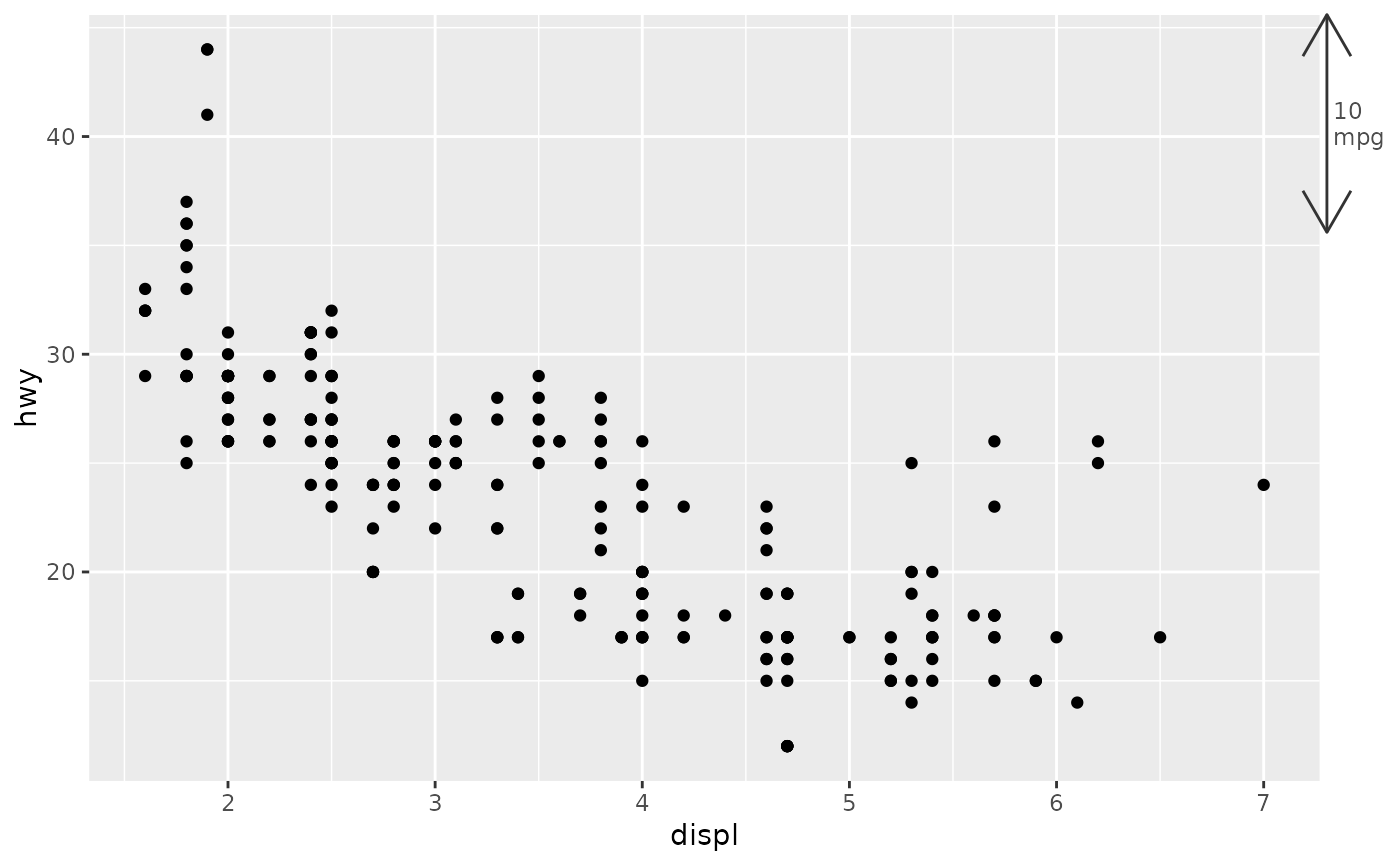This axis guides draws a scale bar to indicate a distance rather than mark absolute values. The function is questioned due to a possible migration of guide functions after ggplot2 releases a new guide system.

## Usage

guide_axis_scalebar(
title = waiver(),
size = NULL,
label = NULL,
colour = NULL,
color = NULL,
just = 1,
position = waiver()
)

## Arguments

title

A character string or expression indicating a title of guide. If NULL, the title is not shown. By default (waiver()), the name of the scale object or the name specified in labs() is used for the title.

size

A numeric(1) for a distance to indicate, in data units. If NULL (default), the median distance between breaks is taken.

label

A character(1) to use as scale bar label. If NULL (default), the size distance is fed to the scale's labeller.

colour, color

A character(1) with a valid colour for colouring the axis text, axis ticks and axis line. Overrules the colour assigned by the theme.

just

A numeric(1) between 0 and 1 to indicate where the scalebar should be drawn relative to the plot panel. The default (1), places is at the right or at the top.

position

Where this guide should be drawn: one of top, bottom, left, or right.

## Value

A axis_scalebar guide class object.

## Details

It is discouraged to use this guide in combination with a scale transformation.

## Theme elements

This axis guide has an alternative understanding of the following theme elements:

axis.ticks.*

An element_line() to draw the scale bar itself.

axis.ticks.length.*

A unit() indicating how far the scale bar should be placed from the plot panel. Can be a negative unit to place the scale bar inside the plot panel.

axis.text.*

The hjust and vjust parameters are used to justify the text along the scale bar, instead of along itself, in the x and y directions respectively.

Other axis-guides: guide_axis_logticks(), guide_axis_manual(), guide_axis_minor(), guide_axis_nested(), guide_axis_truncated()

## Examples

# A standard plot
p <- ggplot(mpg, aes(displ, hwy)) +
geom_point()

# Guide as secondary axis
p + guides(x.sec = "axis_scalebar")# Customising size and label
p + guides(x.sec = guide_axis_scalebar(size = 0.5, label = "0.5 litre"))# Placing the scale bar on top of the plotting panel
p + guides(x.sec = guide_axis_scalebar(just = 0.95)) +
theme(axis.ticks.length.x.top = unit(-2, "lines"))# Adding arrows through the axis.ticks theme element
p + guides(y.sec = guide_axis_scalebar(size = 10, label = "10\nmpg")) +
theme(axis.ticks.y.right = element_line(arrow = arrow(ends = "both")))